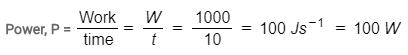Courses

# Test: Conservation Of Energy And Power

## 10 Questions MCQ Test Science Class 9 | Test: Conservation Of Energy And Power

Description
This mock test of Test: Conservation Of Energy And Power for Class 9 helps you for every Class 9 entrance exam. This contains 10 Multiple Choice Questions for Class 9 Test: Conservation Of Energy And Power (mcq) to study with solutions a complete question bank. The solved questions answers in this Test: Conservation Of Energy And Power quiz give you a good mix of easy questions and tough questions. Class 9 students definitely take this Test: Conservation Of Energy And Power exercise for a better result in the exam. You can find other Test: Conservation Of Energy And Power extra questions, long questions & short questions for Class 9 on EduRev as well by searching above.
QUESTION: 1

### What is the commercial unit of energy?

Solution:

The commercial unit of energy is a kilowatt hour.
One kilowatt hour is defined as the amount of electrical energy consumed when an electrical appliance having a power rating of 1 kilowatt is used for 1 hour.

QUESTION: 2

### A stone weighing 1 kg is dropped from rest from a height of 4 metres above the ground. When it has free-fallen 1 metre its total energy with respect to the ground is

Solution:

Energy is conserved in Free - fall situations ( no external forces doing work). Thus, the total energy would be the same at every stage in failing. Whatever total energy body has initially, it will maintain it throughout the course of its motion.
PE = mgh = 1 x 10 x 4 = 40 J
The object begins with 40 J of potential energy and no Kinetic energy. The total mechanical energy (KE + PE) is 40J.

QUESTION: 3

### A lamp consumes 1000 J of electrical energy in 10 seconds. What is its power?

Solution:

Electrical energy consumed by lamp, W = 1000 J
Time taken, t = 10 secondsQUESTION: 4

When we throw a rock from the top of a building which equation describes the energy of the body at each point during the fall?

Solution:

According to the law of conservation of energy , energy can only be transformed from to form to another , it can neither be created nor destroyed . The total energy before and after the transformation always remains constant.

QUESTION: 5

What is not true about energy?

Solution:
QUESTION: 6

If work is done at a faster rate, then

Solution:
QUESTION: 7

A diesel engine supplies 25,000 J of energy in 50 seconds. Power of engine would be

Solution:

Power = Work done / Time
= 25000 / 50
= 500J/s or 500W

QUESTION: 8

If a light bulb is switched on for 20 s and it consumes 2400 J of electrical energy then it's power is

Solution:

Power = Energy /Time

Power = 2400/20

Power = 120w

QUESTION: 9

A ball is thrown vertically upwards. Its velocity keeps on decreasing. What happens to its kinetic energy when its reaches the maximum height?

Solution:

When a body is thrown vertically upward its velocity continuously decreases, kinetic energy also decreases and potential energy increases due to increase in height.

QUESTION: 10

In winters, rubbing of hands together for some time, causes a sensation of warmth mainly because of

Solution:

If you rub your hands together for several seconds, you'll notice that your hands feel warm. That warmth is caused by a force called friction. When objects like your hands come in contact and move against each other, they produce friction. Friction happens when you overcome the resistance of one object rubbing against the other. The force of the friction is opposes the direction of the motion. If you just put your hands together, there's no resistance, so there's no friction produced. Rub them together and there's friction.